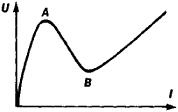# Negative Resistance

(redirected from Negative dynamic resistance)
Also found in: Dictionary.

## negative resistance

[′neg·əd·iv ri′zis·təns]
(electronics)
The resistance of a negative-resistance device.
McGraw-Hill Dictionary of Scientific & Technical Terms, 6E, Copyright © 2003 by The McGraw-Hill Companies, Inc.
The following article is from The Great Soviet Encyclopedia (1979). It might be outdated or ideologically biased.

## Negative Resistance

a property of certain electric circuit elements that is manifested by a reduction of the voltage drop U across the elements when the current I flowing through them is increased (or vice versa). Negative resistance is defined as the quantity.

R- = (ΔUI) < 0

An element with a negative resistance does not consume electric energy but supplies such energy to the circuit; that is, it is an active element. This situation arises because the element contains a source that supplements the energy supply of the circuit.

A negative resistance can be obtained only in a certain region of current and voltage values; outside of that region, (ΔUU) > 0. A negative resistance indicates the existence of a decreasing section AB in the element’s volt-ampere characteristic (see Figure 1).Figure 1

The nature of negative resistance is different in different active elements. If the absolute value of the negative resistance in an element is less than the sum of the positive resistances in the other elements of a circuit, the negative resistance simply provides partial compensation for the circuit losses. If the negative resistance exceeds this sum, the state of the circuit is unstable; there can occur a transition to a state of stable equilibrium or the production of oscillations.

V. V. MIGULIN